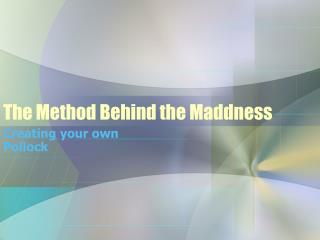DownloadDownload Presentation# The Method Behind the Maddness

Download Presentation## The Method Behind the Maddness

- - - - - - - - - - - - - - - - - - - - - - - - - - - E N D - - - - - - - - - - - - - - - - - - - - - - - - - - -
##### Presentation Transcript

2. Introduction • Jackson Pollock is best known for his works that tie into Fractal Expressionism • After his paintings were analyzed using the box counting method, it was discovered that his paintings were loosely Fractal • Though his style of painting was in no way uniformed or controlled, the final product resembled some uniformity and similarities across the canvas from multiple perspectives

3. Jackson Pollock – Blue Poles

4. Creating my own Pollock • Create a Java applet program that implements a fractal art form loosely similar to the works of Jackson Pollock • This is accomplished by coding the program to draw a large number of random lines using random colors from a given color scheme. • This will be accomplished using a series of for loops and switch statements.

5. Beginning Phases Jackson Pollock Pollock Applet

6. More Pollock Applet vs Pollock Applet Applet Applet Jackson Pollock • Notice the depletion of white space as more random lines are drawn in • Though the applet is similar to Pollock’s painting, it is not exactly fractal

7. Defining fractals WHAT IS A FRACTAL? A fractal is an object that displays self-similarity at various scales. In other words, if we zoom in any portion of a fractal object, we will notice the smaller section is actually a scaled-down version of the big one.

8. The concept of Orbit Fractal Though orbit Fractal generation differ from the traditional fractal generation, it is still fractal by the nature because of iteration process of the same functions. The principal idea of these fractals is the process of tracing out orbits of some function. In this case an initial seed point is chosen instead of an initial shape or line. This seed is then fed into a formula which returns a new point. The new point is plotted and then it is fed into the formula. This process can continue indefinitely or for a user specified number of points. When these points are plotted we find that they converge onto an attractor. This attractor is the fractal itself. The formulas used are of the form: newX := a[k]*x + b[k]*y + e[k] newY := c[k]*x + d[k]*y + f[k] where k is the specific formula.

9. Examples • Orbit Fractals • Traditional Fractals

10. Deriving the Fractals • For this particular fractal (known as Fractal Popcorn), the functions are: • xn + 1 = xn - h * sin(yn + tan(3yn)) • yn + 1 = yn - h * sin(xn + tan(3xn)) • For this fractal, the value of h is set at 0.0025. The iterative process is applied to every tenth pixel, with each iteration being plotted. The default number of iterations is 500

11. Final Result